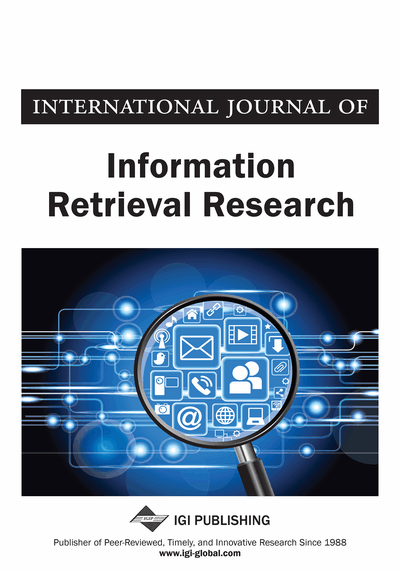# Improved Parameterless K-Means: Auto-Generation Centroids and Distance Data Point Clusters

Wan Maseri Binti Wan Mohd (University Malaysia Pahang, Malaysia), A.H. Beg (University Malaysia Pahang, Malaysia), Tutut Herawan (University Malaysia Pahang, Malaysia), A. Noraziah (University Malaysia Pahang, Malaysia) and K. F. Rabbi (University Malaysia Pahang, Malaysia)
Copyright: © 2011 |Pages: 14
DOI: 10.4018/ijirr.2011070101
OnDemand PDF Download:
Available
\$37.50
No Current Special Offers

## Abstract

K-means is an unsupervised learning and partitioning clustering algorithm. It is popular and widely used for its simplicity and fastness. K-means clustering produce a number of separate flat (non-hierarchical) clusters and suitable for generating globular clusters. The main drawback of the k-means algorithm is that the user must specify the number of clusters in advance. This paper presents an improved version of K-means algorithm with auto-generate an initial number of clusters (k) and a new approach of defining initial Centroid for effective and efficient clustering process. The underlined mechanism has been analyzed and experimented. The experimental results show that the number of iteration is reduced to 50% and the run time is lower and constantly based on maximum distance of data points, regardless of how many data points.
Article Preview
Top

## Introduction

Data clustering is often used in a variety of applications, such as Vector Quantization (VQ) (Lai et al., 2002), pattern recognition (Theodoridis & Koutroumbas, 2003), knowledge discovery (Fayyad et al., 1996), pattern recognition (Liu & Kubala, 2004), debugging and web / data mining. Among the clustering formulations that minimize a cost function, k-means algorithm is possibly the most widely used and studied (Kanungo et al., 2002). It is also known as the Generalized Lloyd Algorithm (GLA) is a special case of a generalized hard clustering scheme, when representatives of section are adopted and squared Euclidean distances are used to measure the distortion (the difference) between a vector X and its representative cluster (cluster center) C (Lai & Huang, 2010).

Existing clustering algorithms can be merely divided into two categories: hierarchical clustering and partional clustering. Classic algorithm K-Means (KM) clustering algorithm is the most popular for its simplicity and efficiency. Although the k-means algorithm is widely used to solve problems in many areas and very sensitive to initialize, the better centers have been chosen, the better results have found. Furthermore, it is easily trapped in local optimal. Recently, various work has been done to overcome these problems (Khan & Ahmad, 2004; Jiang et al., 2010).

Different approaches have been proposed for improving k-means algorithm, which gradually are more successful. These progressive approaches to the cluster are calculated by solving all the problems of intermediate clustering (Bagirov, 2008; Hansen et al., 2005; Likas et al., 2003). The global k-means algorithm has been proposed in Likas et al. (2003) and modified global k-means algorithm has been proposed in Bagirov (2008) and Bagirov and Mardaneh (2006) are gradually clustering algorithms. Bagirov (2008) presented a new version of the global k-means algorithm. The numerical experimental result shown that these algorithms allowed to find a global or near global clusters minimize (or error) function. These algorithms are memory intensive because they require storage of the affinity matrix. Alternatively, this matrix can be calculated each iteration, but would extend the computing time significantly (Bagirov et al., 2011).

K-Means (MacQueen, 1967) is one of the simplest unsupervised learning algorithms to solve the well-known clustering problem. The procedure is simple and easy to classify a data set through a certain number of clusters (assume k clusters) fixed a priority. The main objective is to define k centroids, one for each cluster. These centroids should be placed strategically because different location causes different results. So the best option is to place them as much as possible from each other. The next step is to take each data point and assign it to the nearest center centroid. When all points are assigned, the first step has been completed. At this point have to re-calculate k new centroids of the clusters resulting from the previous step. After that it has these k new centroids, a new binding has to done between the same set of data sets and the newest centroid. The process has been repeated. As a result of this loop, it notes that k Centroid change their location step by step until centroid does not move. Finally, this algorithm is to minimize an objective function, in this case, a-squared error function. The objective function, J is defined as in formula (1).

(1)

The algorithm is composed of the following steps:

• i.

Place K points into the space represented by the objects that are being clustered. These points represent initial group centroids.

• ii.

Assign each object to the group that has the closest centroid.

• iii.

When all objects have been assigned, recalculate the positions of the K centroids.

• iv.

Repeat Steps 2 and 3 until the centroids no longer move. This produces a separation of the objects into groups from which the metric to be minimized can be calculated.

## Complete Article List

Search this Journal:
Reset
Open Access Articles: Forthcoming
Volume 12: 4 Issues (2022): Forthcoming, Available for Pre-Order
Volume 11: 4 Issues (2021): 3 Released, 1 Forthcoming
Volume 10: 4 Issues (2020)
Volume 9: 4 Issues (2019)
Volume 8: 4 Issues (2018)
Volume 7: 4 Issues (2017)
Volume 6: 4 Issues (2016)
Volume 5: 4 Issues (2015)
Volume 4: 4 Issues (2014)
Volume 3: 4 Issues (2013)
Volume 2: 4 Issues (2012)
Volume 1: 4 Issues (2011)
View Complete Journal Contents Listing